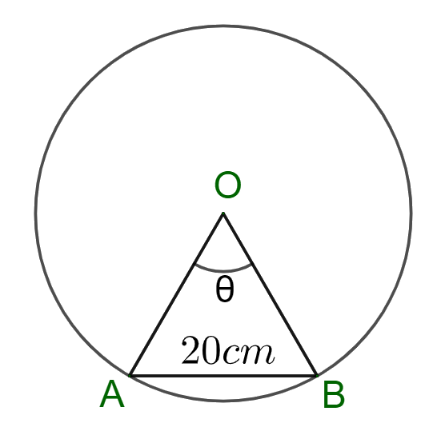# NCERT Solutions For Class 11 Maths Chapter 3 Exercise 3.1

## Chapter 3 Ex.3.1 Question 1

Find the radian measures corresponding to the following degree measures:

(i) $$25^\circ$$

(ii) $$-47^{\circ}$$ $$30^\prime$$

(iii) $$240^{\circ}$$

(iv) $$520^{\circ}$$

### Solution

We know that $$180^\circ = \pi$$ radian

Therefore,

(i) $$25^\circ$$

\begin{align}25^\circ &= \frac{\pi }{180} \times 25 \quad \text{radian }\\&= \frac{5\pi }{36} \quad \text{radian }\end{align}

(ii) $$-47^{\circ}$$ $$30^\prime$$

\begin{align} - 47^\circ 30\prime &= - \left( {47\frac{1}{2}} \right)^\circ = - \left( {\frac{{95}}{2}} \right)^\circ\\- \left( {\frac{{95}}{2}} \right)^\circ &= \frac{\pi }{{180}} \times - \left( {\frac{{95}}{2}} \right)\quad \text{radian}\\&= - \frac{19\pi }{72} \quad \text{ radian}\end{align}

(iii) $$240^{\circ}$$

\begin{align}240^\circ &= \frac{\pi }{{180}} \times 240\quad \text{radian}\\&= \frac{{3\pi }}{4} \quad \text{radian}\end{align}

(iv) $$520^{\circ}$$

\begin{align}520^\circ &= \frac{\pi }{180} \times 520 \quad \text{radian}\\ &= \frac{26\pi }{9} \quad \text{ radian} \end{align}

## Chapter 3 Ex.3.1 Question 2

Find the degree measures corresponding to the following radian measures \begin{align}\left( {\rm{Use}\;\pi = \frac{22}{7}} \right).\end{align}

(i) $$\frac{11}{16}$$

(ii) $$-4$$

(iii) $$\frac{5\pi }{3}$$

(iv) $$\frac{7\pi }{6}$$

### Solution

We know that $$180^\circ = \pi$$ radian

Therefore,

(i) $$\frac{11}{16}$$

\begin{align}\frac{11}{16} \; \text{radian} &= \frac{180^\circ }{\pi } \times \frac{11}{16}\\ &=180\times \frac{7}{22}\times \frac{11}{16}\deg \\ & =\frac{315}{8}\deg \\ & =39\frac{3}{8}\deg \\ & =39\deg +\frac{3}{8}\times 60\min \qquad \quad\left[ \because 1{}^\circ =6{0}' \right] \\ & =39\deg +22\frac{1}{2}\min \\ & =39\deg +22\min +\frac{60}{2}\sec \qquad \left[ \because {1}'=6{0}'' \right] \\ & =39{}^\circ 2{2}'3{0}'' \end{align}

(ii) $$-4$$

\begin{align}-4\; \text{radian} &= \frac{180^\circ }{\pi } \times \left( { - 4} \right)\\ & =180\times \frac{7}{22}\times \left( -4 \right)\deg \\ & =-\frac{2520}{11}\deg \\ & =-229\frac{1}{11}\deg \\ & =-229\deg +\frac{60}{11}\min \qquad \qquad \left[ \because 1{}^\circ =6{0}' \right] \\ & =-22\deg +5\frac{5}{11}\min \\ & =-22\deg +5\min +\frac{5}{11}\times 60\sec \qquad \left[ \because {1}'=6{0}'' \right] \\ & =-22{}^\circ {5}'2{7}'' \\ \end{align}

(iii) $$\frac{5\pi }{3}$$

\begin{align}\frac{{5\pi }}{3}\;\text{radian} &= \frac{{180^\circ }}{\pi } \times \frac{{5\pi }}{3}\\& = 300^\circ \end{align}

(iv) $$\frac{7\pi }{6}$$

\begin{align}\frac{7\pi }{6}\text{ radian}&= \frac{180^\circ }{\pi } \times \frac{7\pi }{6}\\ &= 210^\circ \end{align}

## Chapter 3 Ex.3.1 Question 3

A wheel makes $$360$$ revolutions in one minute. Through how many radians does it turn in one second?

### Solution

A wheel makes $$360$$ revolutions in $$1$$ minute ($$60$$ seconds)

Therefore,

Number of revolutions made by the wheel in $$1$$ second $$= \frac{{360}}{{60}} = 6$$

In one complete revolution, the wheel turns an angle of $$2\pi$$ radians

Hence, in 6 complete revolutions, it will turn an angle of $$6 \times 2\pi = 12\pi$$ radians

Thus, in one second, the wheel turns an angle of $$12\pi$$ radians.

## Chapter 3 Ex.3.1 Question 4

Find the degree measure of the angle subtended at the centre of a circle of radius $$100\, \rm{cm}$$ by an arc of length $$22\,\rm{cm}$$.\begin{align}\left( {\rm{Use}\;\pi = \frac{22}{7}} \right) \end{align}

### Solution

As we know that if in a circle of radius $$r,$$ an arc of length l subtends an angle of $$\rm{\theta}$$ radians,

Then $$l = r\theta$$

Therefore,

\begin{align}\theta &= \frac{l}{r}\text { radian} \\\theta &= \frac{\text{22 cm}}{\text{100 cm}}\text { radian}\\ \theta &=\frac{11}{50}\times \frac{180}{\pi }\deg \\ & =\frac{11}{50}\times 180\times \frac{7}{22}\deg \\ & =\frac{63}{5}\deg \\ & =12\frac{3}{5}\deg \\ & =12\deg +\frac{3}{5}\times 60\min \qquad \left[ \because 1{}^\circ =6{0}' \right] \\ & =12{}^\circ 3{6}'\end{align}

Thus, the required angle is $$12^\circ 36'$$

## Chapter 3 Ex.3.1 Question 5

In a circle of diameter $$40\,\rm{ cm},$$ the length of a chord is $$20 \,\rm{cm}.$$ Find the length of minor arc of the chord.

### Solution

Diameter of the circle$$= 40\,\rm{ cm}$$

Therefore, Radius of the circle $$r = \frac{\rm{40\,cm}}{2} = 20\,\rm{cm}$$

Let $$AB$$ be a chord of length $$20\,\rm{ cm}$$ of the circle.In $$\Delta AOB$$

$$AB = 20cm$$

$$OA = OB = r = 20\,\rm{cm}$$

Hence, $$\Delta AOB$$ is an equilateral triangle

Thus, $$\theta = 60^\circ$$ or $$\theta = \frac{\pi }{3}$$ radian

As we know that if in a circle of radius $$r,$$ an arc of length $$l$$ subtends an angle of $$θ$$ radians,

Then $$l = r\theta$$

Therefore,

\begin{align} l&=r\theta \\ {AB} &=20\,\rm{cm}\times \frac{\pi }{3} \\ & =\frac{20\pi }{3}\,\rm{cm}\end{align}

Hence, the length of the minor arc of the chord is $$\frac{20\pi }{3}\,\rm{cm}$$.

## Chapter 3 Ex.3.1 Question 6

If in two circles, arcs of the same length subtend angles $$60^\circ$$ and $$75^\circ$$ at the centre, find the ratio of their radii.

### Solution

Let the radii of the two circles be $$r$$ and $$R.$$

Let an arc of length l subtend an angle of $$60^\circ$$ at the centre of the circle of radius $$r,$$ and $$75^\circ$$ at the centre of the circle of radius $$R.$$

Now,

$$60^\circ = \frac{\pi }{3}$$ radian and $$75^\circ = \frac{{5\pi }}{{12}}$$ radian

As we know that if in a circle of radius $$r,$$ an arc of length $$l$$ subtends an angle of $$θ$$ radians,

Then $$l = r\theta$$

Therefore,

\begin{align}l &= r \times \frac{\pi }{3}\\ &= \frac{{\pi r}}{3}\end{align} and \begin{align}l &= R \times \frac{5\pi }{12}\\ &= \frac{5\pi R}{12}\end{align}

Thus,

\begin{align}\frac{{\pi r}}{3} &= \frac{{5\pi R}}{{12}}\\\frac{r}{R} &= \frac{5}{4}\\r:R &= 5:4\end{align}

Hence, the ratio of their radii is $$5:4$$.

## Chapter 3 Ex.3.1 Question 7

Find the angle in radian through which a pendulum swings if its length is $$75\,\rm{ cm}$$ and the tip describes an arc of length

(i) $$10\,\rm{cm}$$

(ii) $$15\, \rm{cm}$$

(iii) $$21\,\rm{ cm}$$

### Solution

As we know that if in a circle of radius $$r,$$ an arc of length $$l$$ subtends an angle of $$θ$$ radians,

Then $$l = r\theta$$

(i) Radius, $$r = 75 \,\rm{cm}$$ and length of the arc, $$l = 10 \rm{cm}$$

\begin{align}\theta &= \frac{l}{r}\\ &= \frac{\rm{10cm}}{\rm{75\,cm}}\\ &= \frac{2}{15}\end{align}

Thus, $$\theta = \frac{2}{{15}}$$ radian

(ii) Radius, $$r = 75\,\rm{cm}$$ and length of the arc, $$l = 15\,\rm{cm}$$

\begin{align}\theta &= \frac{l}{r}\\ &= \frac{\rm{15\,cm}}{\rm{75\,cm}}\\ &= \frac{1}{5}\end{align}

Thus, $$\theta = \frac{1}{5}$$ radian

(iii) Radius, $$r = 75\,\rm{cm}$$ and length of the arc, $$l = 21\,\rm{cm}$$

\begin{align}\theta &= \frac{l}{r}\\ &= \frac{\rm{21\,cm}}{\rm{75\,cm}}\\ &= \frac{7}{{25}}\end{align}

Thus, $$\theta = \frac{7}{25}$$ radian

Instant doubt clearing with Cuemath Advanced Math Program